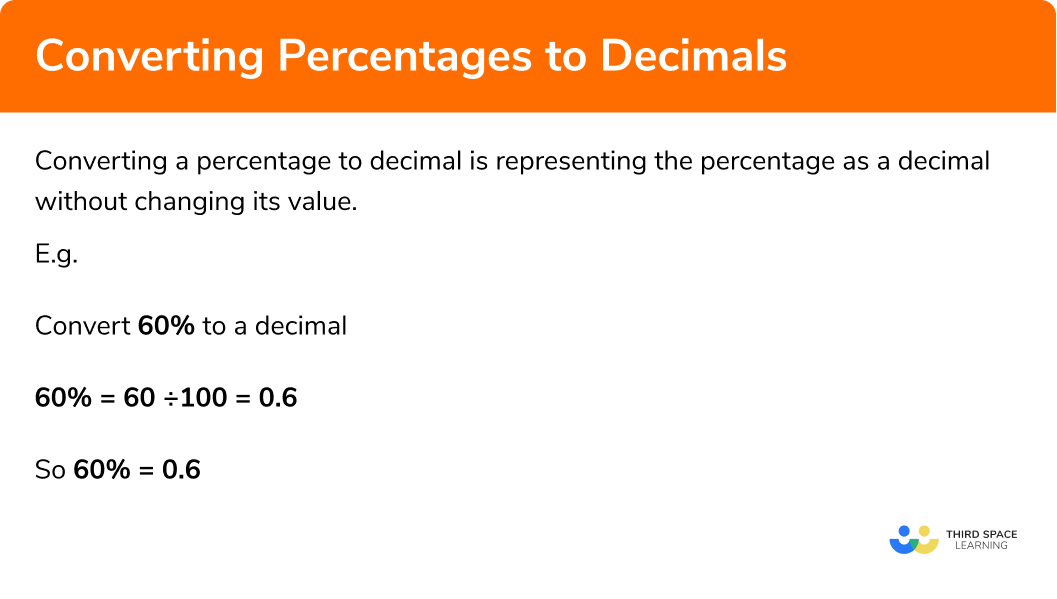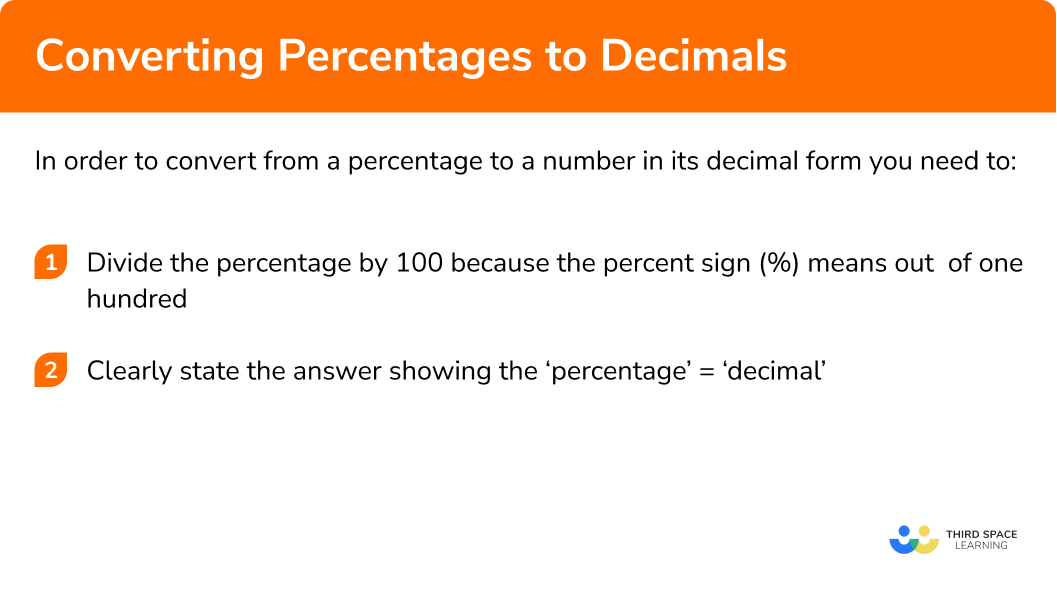GCSE Maths Number FDP Converting FDP

Percentage To Decimal

# Percentage To Decimal

Here we will learn about converting percentages to decimals.
There are also converting percentages to decimals worksheets based on Edexcel, AQA and OCR exam questions, along with further guidance on where to go next if you’re still stuck.

## What is converting percentages to decimals?

Converting a percentage to decimal is representing the percentage as a decimal without changing its value.

E.g.

\begin{aligned} &25\%=0.25\\\\ &45 \%=0.45\\\\ &33.\dot{3} \%=0.33333 \ldots\\\\ &80 \%=0.8 \end{aligned}

### What is converting percentages to decimals?## How to convert percentages to decimals

In order to convert from a percentage to a number in its decimal form you need to:

1. Divide the percentage by \pmb{100} because the percent sign (%) means out of one hundred.
2. Clearly state the answer showing the ‘percentage’ = ‘decimal’.

### Explain how to convert a percentage to a decimal in 2 stepsTo learn how to convert decimals to percentages check out:

Step by step guide: Decimal to Percentage

## Converting percentages to decimals examples

### Example 1: converting a simple percentage to a decimal

Convert 60% to a decimal.

1. Divide the percentage by a hundred since the percent sign (%) means out of \pmb{100}.

You know that the percentage sign (%) means the number is out of 100. Therefore if we divide the number by a hundred we will have the equivalent decimal.

$60\% = 60\div100=0.6$

When we divide by 100 we move the digits two places to the right.
The 6 has moved from the tenths column to the tenths column in the first decimal place.
The 0 has moved from the units column (ones column) to the hundredths column in the second decimal place.

The decimal point does not move.

2Clearly state the answer showing the ‘percentage’ = ‘decimal’.

$60\% = 0.6$

### Example 2: converting a simple percentage to a decimal

Convert 8% to a decimal.

$8\% = 8\div100=0.08$

$8\% = 0.08$

### Example 3: converting a percentage (involving a decimal) to a decimal

Convert 52.3% to a decimal.

$52.3\% = 52.3\div100=0.523$

$52.3\% = 0.523$

### Example 4: converting a percentage (involving a recurring decimal) to a decimal

Convert 4.\dot{2} to a decimal.

$4.\dot{2}\% = 4.\dot{2}\div100$

Note: Remember the 2 is a recurring decimal. It will still be recurring indefinitely after being divided by 100.

E.g.

$0.\dot{2}\div100= 0.00\dot{2}$

Therefore,

$4.\dot{2}\% = 4.\dot{2}\div100= 0.04\dot{2}$

$4.\dot{2}\% = 0.04\dot{2}$

### Example 5: converting a percentage (above 100) to a decimal

Convert 145.1% to a decimal.

$145.1\% = 145.1\div100=1.451$

$145.1\% = 1.451$

### Example 6: converting a percentage to a decimal

Convert 102.04% to a decimal.

$102.04\% = 102.04\div100=1.0204$

$102.04\% = 1.0204$

### Calculator skills

Convert 12% to a decimal.

1. Insert the value of the percentage into your calculator.
2. Divide by \pmb{100}.
3. Press the = button. Here your calculator will give the answer as a fraction in its simplest form.
4. Press the  \pmb{[s ⇔ d]} button to have it shown as a decimal.

12% = 0.12

### Common misconceptions

• Multiplying incorrectly by \pmb{100}

Often mistakes are made when dividing by 100. The common mistakes are:

− Dividing by 10 not 100.

− Making mistakes with decimals.

Make sure you are confident with the below calculations:

E.g.

\begin{aligned} &4 \div 100=0.04 \\\\ &0.4 \div 100=0.004 \\\\ &0.04 \div 100=0.0004 \end{aligned}

• Recurring decimals in the percentages

You need to remember to take the recurring decimal into account when multiplying by an order of 10.

E.g.

$0.\dot{4}\times10=4.\dot{4} \; \text{not} \; 4.4$

Percentage to decimal is part of our series of lessons to support revision on comparing fractions, decimals and percentages. You may find it helpful to start with the main comparing fractions, decimals and percentages lesson for a summary of what to expect, or use the step by step guides below for further detail on individual topics. Other lessons in this series include:

### Practice percentage to decimal questions

1. Convert 25\% to a decimal

250.2542.525\% means 25 ÷ 100 which is equal to 0.25

2. Convert 87.5\% to a decimal

1.\dot{1}4285\dot{7}87.50.8758.7587.5\% means 87.5 ÷ 100 which is equal to 0.875

3. Convert 175\% to a decimal

1.750.17517517.5175\% means 175 ÷ 100 which is equal to 1.75

4. Convert 760\% to a decimal

7600.767.357.6760\% means 760 ÷ 100 which is equal to 7.6

5. Convert 44. \dot{4}\% to a decimal

0.40.440.4440.\dot{4}44.\dot{4}\% means 44.\dot{4} ÷ 100 which is equal to 0.\dot{4}

6. Convert 18.\dot{1}\dot{8}\% to a decimal

0.180.1818180. \dot{1}\dot{8}1. \dot{8}\dot{1}18.\dot{1}\dot{8}\% means 18.\dot{1}\dot{8} ÷ 100 which is equal to 0. \dot{1}\dot{8}

### Percentage to decimal GCSE questions

1.

a)  Convert 52\% to a decimal.

b)   Convert 25\% to a decimal.

c)  Convert 80\% to a decimal.

(3 marks)

a) 0.52

(1)

b) 0.25

(1)

c) 0.8

(1)

2.

a)  Convert 250\% to a decimal.

b)   Convert 160\% to a decimal.

c)  Convert 20\% to a decimal.

(3 marks)

a) 2.5

(1)

b) 1.6

(1)

c) 0.2

(1)

3.

a)  Convert 70\% to a decimal.

b)   Convert 8\% to a decimal.

c)  Convert 22. \dot{2}\% to a decimal.

(3 marks)

a) 0.7

(1)

b) 0.08

(1)

c) 0.\dot{2}

(1)

4.

a)  Convert 101\% to a decimal.

b)   Convert 16.\dot{6}\% to a decimal.

(2 marks)

a) 1.01

(1)

b) 0.1\dot{6}

(1)

## Learning checklist

You have now learned how to:

• Convert percentages to a decimal
• Convert percentages (with a decimal) to a decimal
• Convert percentages (with a recurring decimal) to a decimal

## Still stuck?

Prepare your KS4 students for maths GCSEs success with Third Space Learning. Weekly online one to one GCSE maths revision lessons delivered by expert maths tutors.

Find out more about our GCSE maths tuition programme.